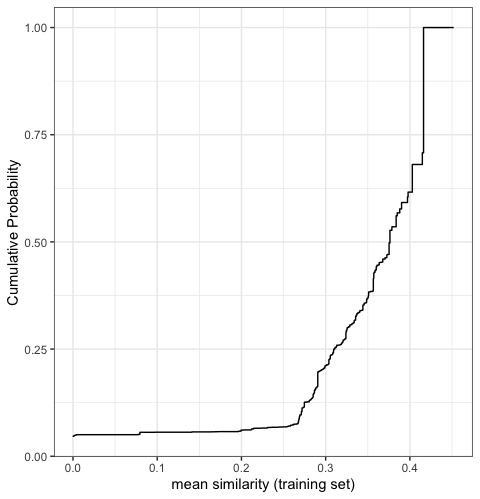Applicability domain methods for binary data

Introduction

library(applicable)

Similarity statistics can be used to compare data sets where all of the predictors are binary. One of the most common measures is the Jaccard index.

For a training set of size n, there are n similarity statistics for each new sample. These can be summarized via the mean statistic or a quantile. In general, we want similarity to be low within the training set (i.e., a diverse training set) and high for new samples to be predicted.

To analyze the Jaccard metric, applicable provides the following methods:

• apd_similarity: analyzes samples in terms of similarity scores. For a training set of n samples, a new sample is compared to each, resulting in n similarity scores. These can be summarized into the median similarity.

• autoplot: shows the cumulative probability versus the unique similarity values in the training set.

• score: scores new samples using similarity methods. In particular, it calculates the similarity scores and if add_percentile = TRUE, it also estimates the percentile of the similarity scores.

Example

The example data is from two QSAR data sets where binary fingerprints are used as predictors.

data(qsar_binary)

Let us construct the model:

jacc_sim <- apd_similarity(binary_tr)
jacc_sim
#> Applicability domain via similarity
#> Reference data were 67 variables collected on 4330 data points.
#> New data summarized using the mean.

As we can see below, this is a fairly diverse training set:

library(ggplot2)

# Plot the empirical cumulative distribution function for the training set
autoplot(jacc_sim)We can compare the similarity between new samples and the training set:

# Summarize across all training set similarities
mean_sim <- score(jacc_sim, new_data = binary_unk)
mean_sim
#> # A tibble: 5 x 2
#>   similarity similarity_pctl
#>        <dbl>           <dbl>
#> 1     0.376            49.8
#> 2     0.284            13.5
#> 3     0.218             6.46
#> 4     0.452           100
#> 5     0.0971            5.59

Samples 3 and 5 are definitely extrapolations based on these predictors. In other words, the new samples are not similar to the training set and so predictions on them may not be very reliable.